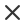FiltrTyp média
Typ licence
Orientace
Styl
Neobsahuje

# Mathematical Operation Reklamní vektory a ilustrace

Physics formulas mathematical equations physics theories arithmetic calculations blackboard with scientific formulas vector background education learning at university or schoolMathematics education and school lesson concept textbook or notebook with writings calculator pen and compass around of green chalkboard with tasks and math formulas cartoon vector illustrationMath formulas mathematical formulas on green school chalkboard handwritten scientific math equations theories or calculations vector background algebra and geometry education conceptMathematical vector seamless pattern with mathematical figures formulas and equations shuffled together you can use any color of backgroundHand drawn math symbols icon in flat style mathematics formula vector illustration on isolated background school education sign business conceptMath education vector pattern with handwritten formulas tasks plots calculations and geometrical figures vector illustrationSchool chalk symbols icon set numbers letters and mathematical symbols green blackboard vector illustration flat designPhysical formulas and phenomenon hand drawn illustration science board with math physics education at school vector illustrationLogarithmic derivative trigonometric logarithmic hyperbolic and inverse equations and formulas on a black background in perspectivePhysical vector seamless pattern with the equations figures schemes plots and other calculations you can use any color of backgroundMathematic vector seamless pattern with different signs figures and formulas back to school seamless scientific backgroundMathematical vector seamless pattern with geometrical plots formulas and calculations endless texture you can use any color of backgroundMath educational vector seamless pattern with formulas calculations equations plots and geometrical figures handwritten on grid copybook paper endless mathematical textureAlgebraic identities expression formula doodle handwriting collection set vector illustration scientific education square formulas mathematics resources for teacher and student backgroundMathematical vector seamless pattern with angle protractors triangles drawing rulers formulas and calculations on a green backgroundSchool seamless vector doodle pattern with different mathematical formulas and values good background for school printed supplies stationery other education stuffSeamless background on the topic of mathematical theorems symbols and formulas light contour on a brown backgroundYoung woman sitting on the floor and solving math problem with black board female person dressed in casual clothes study at home vector flat color illustrationA blackboard with algebra formula a contemporary style vector flat design illustration isolated black background square layoutMath formula scientific symbols mathematics infographic graphs numbers and scheme for geometry equations garish vector letters collectionMath chalkboard vector illustration physics solving equation blackboard sketch with geometrical class problem solution or algebra formula higher mathematics intelligence or complex calculation mess